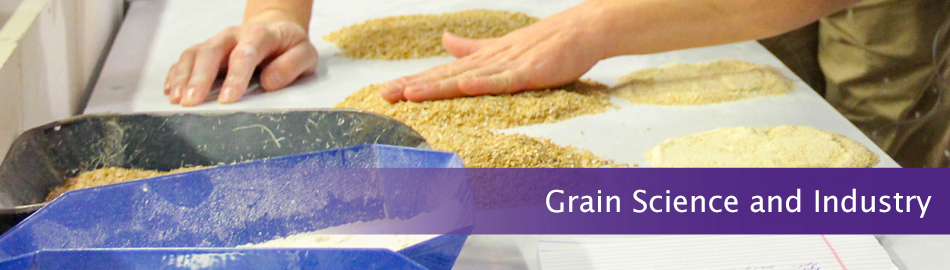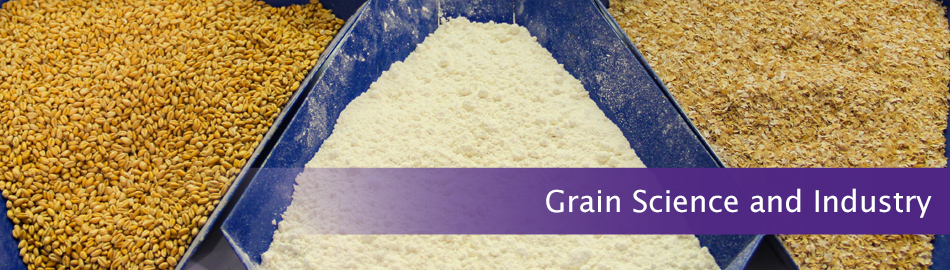# Kansas State University

search

## Grain Science and Industry### Feed Quality Assurance Lab

#### Services Provided

##### •Particle Size Analysis

A sample size of at least 100 grams is needed to perform this analysis. Samples are split using a riffle divider and then weighed out to 100 ± 5 grams of sample. Sieving agent (0.5 grams) will be mixed with the 100 gram sample. Please provide special request if samples need to be ran without sieving agent. Empty sieves are weighed with agitators to determine the empty sieves' weight. The 100 gram sample is then added to the top sieve and placed in the Ro-Tap machine for 10 minutes. Once the test is completed, each sieve is weighed with agitators a second time. The post-test weights are entered into a particle size spread sheet to calculate the geometric mean (dgw) and the geometric standard deviation (Sgw).

##### •Pellet Durability Index
###### Tumblebox Analysis Method

A sample size of at least 1200 grams is needed to perform this analysis. Samples are divided with a riffle divider and sifted with a corresponding sieve. Samples are then weighed to 500 ± 5g of sifted sample. Prepared samples are placed into one compartment of the tumble box. If modified method is selected, 3- 3/4 inch hex nuts will be placed into the compartment with the prepared sample. The machine will run for ten minutes. Once the test is completed, the sample is removed from the compartment. If the modified method was selected, the hex nuts will be removed before the samples are sifted again with the same corresponding sieve. The sample is then weighed a final time. The pellet durability index will be calculated by dividing the finial weight by the initial weight.

###### Holmen 100 Analysis Method

A sample size of at least 400 grams is needed to perform this analysis. Samples are divided with a riffle divider and sifted with a corresponding sieve. Samples are then weighed to 100 ± 5g of sifted sample. The machine will run for 60 seconds, unless a different amount of time is specified. Once the test is completed, the sample is removed, sifted again with the same corresponding sieve, and then weighed a final time. The pellet durability index will be calculated by dividing the finial weight by the initial weight.

##### •Moisture Analysis

A sample size of at least 50 grams is needed to perform this analysis. All samples are ground in preparation for analysis. The moisture pan is weighed prior to addition of any sample material. After noting the empty moisture pan's weight, two grams of sample is added to pan. The sample is then be placed in a moisture drying oven at a specified temperature for a specified time. Once the drying time is complete, the sample is placed in a desiccator for 15 minutes to allow the sample to reach room temperature. The sample is then weighed a final time before the percentage of moisture loss on drying is calculated.

##### •Composite Flow Index
###### Angle of Repose Analysis

A sample size of at least 500 grams is needed to perform this analysis. Samples are riffle divided to obtain the needed amount of test material. Test material is allowed to flow freely over a flat circular platform of a known diameter. The diameter of the platform and height of the pile will be used to calculate the angle of repose.

###### Percentage of Compressibility

Bulk Density

A sample size of at least 500 grams is needed to perform this analysis. Samples are riffle divided to obtain 100 grams of test material. All 100 grams of test material will be placed in a funnel over a 250 mL beaker. Once all the test material is placed into the funnel, the test material will be allowed to flow freely into the beaker. The results are recorded in mL.

Tap Density

A sample size of at least 500 grams is needed to perform this analysis. Samples are riffle divided to obtain 100 grams of test material. All 100 grams of test material is placed in a funnel over a 250 mL beaker. Once all the test material is placed into the funnel, the test material is allowed to flow freely into the beaker. Fifty taps are applied to the beaker while rotating the cylinder. After fifty taps have been applied the results are measured in mL.

###### Critical Orifice Diameter

A sample size of at least 500 grams is needed to perform this analysis. Samples are riffle divided to obtain 50 grams of test material. Test material is placed into a funnel and allowed to free fall into the bin. The test material is allowed to settle in the bin for 30 seconds. After 30 seconds the bin will be opened allowing the test material to fall through the desired disk size. In order for the test material to pass the desired disk size, it must not bridge or rat hole three consecutive times. The sample will then need to fail one disk size smaller.